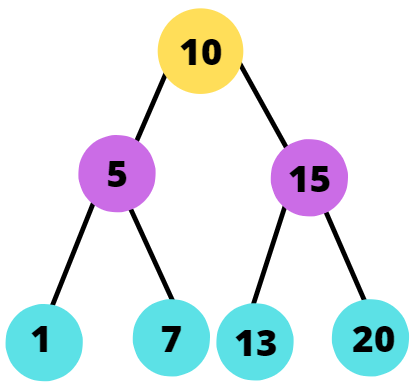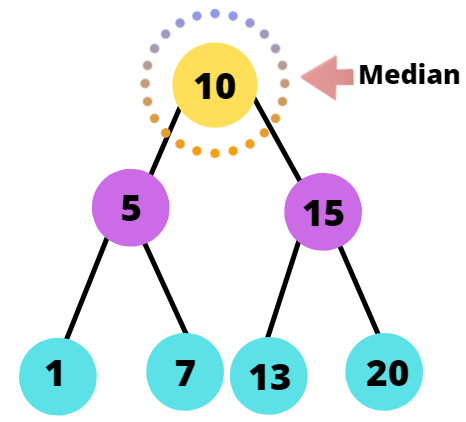# Kth Smallest Element in a Perfect Binary Search Tree

## Introduction

In this question, we are given a perfect binary search tree and an integer K as input. We have to find the Kth smallest element in the perfect binary tree. We will go through two methods to solve this problem.

Before moving on to the solution, we need to keep in mind the two unique properties of the given tree.

1. It is Perfect.
2. It is not an ordinary Binary Tree but a Binary Search Tree.

## Problem Statement

Find the Kth Smallest Element in a Perfect Binary Search Tree

### Test Case

Let us take three different values of K for this tree to understand the problem better.Input: K= 4

Output: 10

Input: K= 2

Output: 5

Input: K= 7

Output: 20

## Method 1: Using Inorder Traversal

An essential property of the Binary Search Tree is that Inorder traversal always prints the keys in sorted ascending order.

In the case of the Inorder Traversal method, we first visit the left subtree, then the root, and finally the right subtree. For our input Perfect Binary Search Tree, the Inorder traversal is,

You can see the keys are sorted in ascending sequence. Now that we have a sorted sequence, we can easily extract the Kth element and print it to get the required answer.

As we saw in Test Cases, If K = 4, the Output should be 10.

Therefore the Kth element in the sequence is also the Kth smallest element of the tree.

So we can solve the problem by,

• doing an inorder traversal and,
• keeping track of no. of nodes visited.

If the number of nodes visited becomes ‘K’, we print the data of the node.

NOTE: Inorder traversal prints keys in sorted ascending order, irrespective of the fact the tree is Perfect or not.

### Steps of algorithm

1. Start Inorder traversal of given Perfect Binary Search Tree
2. While root is not equal to NULL, recursively do the following steps
• Recur for left subtree
• Increase count by 1
• If count == K, return the root’s data
• Recur for right subtree

### Implementation in C++

``````#include <bits/stdc++.h>
using namespace std;
// Structure of Node
struct Node
{
int data;
Node *left, *right;
Node(int x)
{
data = x;
left = right = NULL;
}
};
// Utility function to insert the new nodes
Node *insertNode(Node *node, int data)
{
if (node == NULL)
return new Node(data);
if (data < node->data)
node->left = insertNode(node->left, data);
else if (data > node->data)
node->right = insertNode(node->right, data);
return node;
}
// Inorder traversal of the tree with count
void solve(Node *root, int K, int &count)
{
if (root != NULL)
{
// Recur for left subtree
solve(root->left, K, count);
//Increase count by one
count++;
//If count == K, print root's data
if (count == K)
{
cout << root->data;
return;
}
// Recur for right subtree
solve(root->right, K, count);
}
}
int main()
{
Node *root = NULL;
int K;
// Inserting root node
root = insertNode(root, 10);
//Inserting other nodes
insertNode(root, 5);
insertNode(root, 1);
insertNode(root, 7);
insertNode(root, 15);
insertNode(root, 13);
insertNode(root, 20);
cout<<"Enter the Kth value: "<<endl;
cin>>K;
int count = 0;
cout << "The Kth smallest element is: ";
solve(root, K, count);
cout << endl;
return 0;
}``````

Input:

``````Enter the Kth value:
3``````

Output

``The Kth smallest element is: 7``

#### Complexity Analysis

Time Complexity: O(N), as we are doing an inorder traversal for all the nodes of the tree till count becomes K. In the worst case, K can be equal to N and hence the time complexity is O(N). Here N refers to the number of nodes in the tree.

Space Complexity: O(logN)as we are using recursion in our code. At any moment, the maximum elements in the recursion call stack will be O(logN) (logN refers to the height of the tree). Here N refers to the number of nodes in the tree.

## Method 2: Using median

In this problem, the given Binary Search Tree is perfect and we also know the number of the nodes, i.e., N. We can use these two pieces of information to get a more efficient solution than Method 1 and reduce the time complexity factor from O(N) to O(logN).

The given BST being perfect, will always have an odd number of nodes, i.e., N.

The position of the median for a Perfect BST is given by,

(N+1)/2

In the example tree, the position of the median is 4 using the above formula. The inorder traversal of the same tree is,

1, 5, 7, 10, 13, 15, 20

In the 4th position of the above sequence, 10 is present. So the median of the tree is 10.But how can the median help us in finding the Kth smallest element?

Using the position of the median, we can locate or find out the Kth smallest element.

• If the position of the median is equal to K, it gives the value of Kth smallest element.
• If the position of the median is less than K, it refers to the fact that the Kth smallest element is in the left subtree of the current median.
• If the position of the median is greater than K, it refers to the fact that the Kth smallest element is in the right subtree of the current median.

### Steps of algorithm

1. Find the location of the current median
2. If (K == location_of_median)
• Return the data of median
1. No. of nodes = No. of nodes/2
2. If (K<location_of_median)
• Recur for left subtree with value K
3. If (K>location_of_median)
• K = K - location_of_median
• Recur for right subtree with value K

### Implementation in C++

``````#include <bits/stdc++.h>
using namespace std;
//Structure of Node
struct Node
{
int data;
Node *left;
Node *right;
Node(int x)
{
data = x;
left = right = NULL;
}
};
Node *insertNode(Node *node, int data)
{
if (node == NULL)
return new Node(data);
if (data < node->data)
node->left = insertNode(node->left, data);
else if (data > node->data)
node->right = insertNode(node->right, data);
return node;
}
bool solve(Node *root, int K, int no_of_nodes, int &ans)
{
if (root == NULL)
return false;
//Finding the location of median
int location_of_median = (no_of_nodes + 1) / 2;
//If the current location of the median is equal to K, return ans
if (K == location_of_median)
{
ans = root->data;
return true;
}
//Current no of nodes in the subtree will be half of the total no of nodes of the tree
int current_no_of_nodes = no_of_nodes / 2;
//If K<location_of_median, recur for left subtree
if (K < location_of_median)
return solve(root->left, K, current_no_of_nodes, ans);
//If K>location_of_median, recur for right subtree
K = K - location_of_median;
return solve(root->right, K, current_no_of_nodes, ans);
}
int main()
{
Node *root = NULL;
int K;
// Inserting root node
root = insertNode(root, 10);
//Inserting other nodes
insertNode(root, 5);
insertNode(root, 1);
insertNode(root, 7);
insertNode(root, 15);
insertNode(root, 13);
insertNode(root, 20);
int N = 7;
cout<<"Enter the Kth value: "<<endl;
cin>>K;
int ans = -1;
if (solve(root, K, N, ans))
cout << "The Kth smallest element is: " << ans << endl;
return 0;
}``````

Input:

``````Enter the Kth value:
5``````

Output:

``The Kth smallest element is: 13``

#### Complexity Analysis

Time Complexity: O(logN), as in every step we are going one level deeper in the tree, so we will have to traverse maximum O(logN) nodes every time (logN refers to the height of the tree). Here N refers to the number of nodes in the tree.

Space Complexity:O(logN)as we are using recursion in our code. At any moment, the maximum elements in the recursion call stack will be O(logN) (logN refers to the height of the tree). Here N refers to the number of nodes in the tree.

Q1 What is inorder traversal of a tree?

Ans: Inorder traversal of a tree is one of the three popular ways to traverse any tree. In the case of Inorder traversal, we traverse the left subtree first, then the root, and finally the right subtree.

If the given tree is a Binary Search Tree, then inorder traversal of the tree will always sort the nodes in ascending/ increasing order.

Q2 How to find the median in a Binary Search Tree?

Ans: For a Binary Search Tree with even nodes,

median = (N/2th Node+ (N+1)/2th Node)/2th Node

and for odd nodes,

median = (N+1)/2th Node

Here N refers to the number of nodes in the Binary Search Tree.

Q3 What is a Binary Search Tree?

Ans: A Binary Search Tree is a special kind of Binary Tree, where every node to the left of the root is smaller than the root, and every node to the right of the root is greater than the root. Every subtree of a binary search tree also needs to be a binary search tree.

Q4 What is a Perfect Binary Search Tree?

Ans: A Perfect Binary Search Tree is a Binary Search Tree in which every internal node has exactly two children. Additionally, all the leaf nodes also have to be at the same level.

## Key Takeaways

In this blog, we learned how to approach and solve this question, “Kth Smallest Element in a Perfect Binary Search Tree” in this blog. We saw the Naive Approach with O(N) time complexity and then the more efficient solution with O(logN) time complexity, which exploits the fact that the given BST is perfect.

We hope you got to learn something new and valuable from this blog.

Happy learning, Ninja!